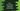# C++ program to find the first and the last occurrence of a character in a string# First and last occurrence of a character in a string in C++ :

Let’s find out the first and the last occurrence of a user given character in a string in C++. C++ provides two methods to do that easily, but I will also show you how to solve it by using a loop. The program will take the string and the character as input from the user, finds out the first and the last occurrence position and print these values.

## Using a loop :

``````#include <iostream>
using namespace std;

int main()
{
// 1
string str;
char c;

// 2
cout << "Enter the string : " << endl;
getline(cin, str);
cout << "Enter the character : " << endl; cin >> c;

// 3
int startIndex = -1;
int endIndex = -1;

// 4
for (int i = 0; i < str.length(); i++)
{
// 5
if (str[i] == c)
{
if (startIndex == -1)
{
startIndex = i;
}
endIndex = i;
}
}

// 6
if (startIndex == -1)
{
cout << "The character is not found in the string." << endl;
}
else
{
cout << "First occurrence : " << startIndex + 1 << endl;
cout << "Last occurrence : " << endIndex + 1 << endl;
}
return 0;
}``````

### Explanation :

The commented numbers in the above program denote the step numbers below :

1. Create two variables str and c. str is to store the string and c is to store the character.
2. Ask the user to enter the string and the character. Read both and store them in str and c.
3. Create two integer variables startIndex and endIndex to store the start and the end indices of the user input character. Initialize them with value -1.
4. Run one for loop to iterate through the characters one by one.
5. Inside the loop, check if the current character is equal to the user-provided character or not. If both are equal, update both startIndex and endIndex. Update startIndex only if its value is not -1 i.e. the character is not found before.
6. Finally, check the value of startIndex. If it is -1, i.e. the indices are not updated or the character is not found in the string. Else, print out the values of startIndex and endIndex. Index in a string starts from 0. Add 1 to print the actual position.

### Sample Examples :

``````Enter the string :
Hello world
Enter the character :
x
The character is not found in the string.

Enter the string :
Hello world
Enter the character :
o
First occurrence : 5
Last occurrence : 8``````

## Using strchr and strrchr :

strchr and strrchr methods are used to find the first and the last occurrence of a character in a string. strchr returns the pointer to the first occurrence of a character and strrchr returns the pointer to the last character of a string. Note that both take c type string.

``````#include <iostream>
#include <string>
using namespace std;

int main()
{
string str;
char c;

cout << "Enter the string : " << endl;
getline(cin, str);

cout << "Enter the character : " << endl; cin >> c;

int startIndex = -1;
int endIndex = -1;

const char *pfirst;
pfirst = strchr(str.c_str(), 'o');
startIndex = pfirst - str.c_str();

const char *plast;
plast = strrchr(str.c_str(), 'o');
endIndex = plast - str.c_str();

if (startIndex == -1)
{
cout << "The character is not found in the string." << endl;
}
else
{
cout << "First occurrence : " << startIndex + 1 << endl;
cout << "Last occurrence : " << endIndex + 1 << endl;
}

return 0;
}``````

The integer variables startIndex and endIndex are used to store the first and last occurrence of the character. We are converting the C++ string to c type string using the cstr()_ method.

The output will be the same as the above example.